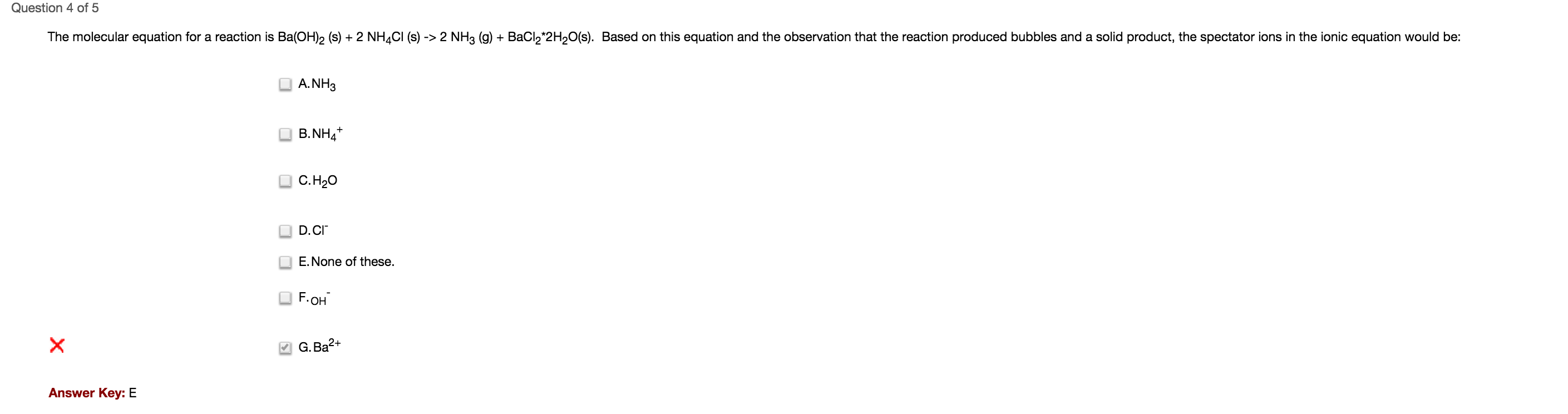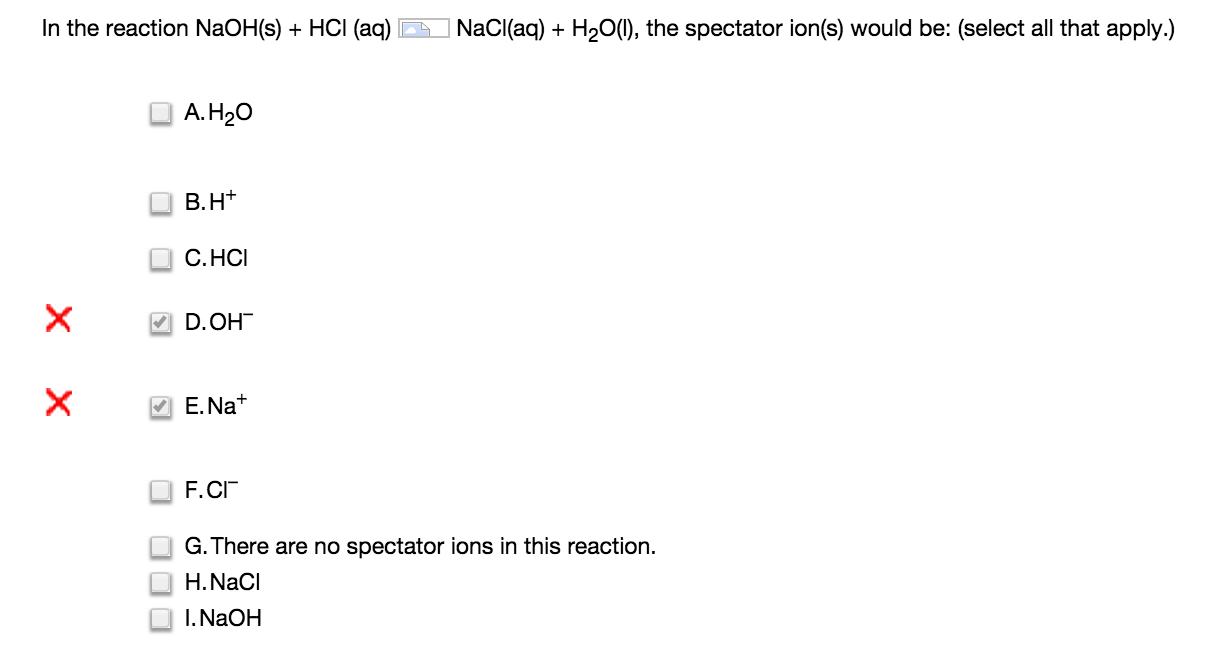```Studying for Chemistry Lab Final

Count sig figs for all numbers involved (just use logic), then round to the least of them for any operation (add/sub/mult/div).

The molecular weight of substance is = number of grams of a mole of that substance. So 1 mole of oxygen is 16 grams (around twice its atomic number). 1 mole of hydrogen is 1 gram.

Calorimetry: heat transfer measurement.

High pH means very basic, low pH means very acidic.

The rocket reaction was generating water.

atoms in a mole of substance: 6.022*10^23

Only break up aqueous to create the net ionic equation. Hydrogens out in front usually come off, go positive. Usually the first part is + and the second part is -.

Spectator ions are ones that are in ion form in both sides (so they should have to be within an aqueous substance).

pH meter measures how many H+’s there are. The more there are, the more basic.

q_sol: mass*specific heat*temperature change.

q_rxn: -(q_soln + q_cal)… (negative change in temp means positive heat of reaction)

H_rxn just means calculating the heat of formation of each of the products and reactants and then taking the difference. Hess’ law just means taking many and adding them all together to show they’re equivalent to their components.

Energy of wave: E=h*v, where h is planks constant.

Large wavelength —> low energy (duh)

How to know *cry:￼￼
￼

Main things to remember:

atoms in a mole of substance: 6.022*10^23

Only break up aqueous to create the net ionic equation. Hydrogens out in front usually come off, go positive. Usually the first part is + and the second part is -.

pH meter measures how many H+’s there are. The more there are, the more basic.

q_sol: mass*specific heat*temperature change.

q_rxn: -(q_soln + q_cal)… (negative change in temp means positive heat of reaction)

H_rxn just means calculating the heat of formation of each of the products and reactants and then taking the difference. Hess’ law just means taking many and adding them all together to show they’re equivalent to their components.

Energy of wave: E=h*v, where h is planks constant. Remember speed of light constant, so c = wavlen * velocity (to find velocity).

```